In organic chemistry, it is important to determine the state of the electrons. This is because the movement of electrons causes reactions to occur between organic compounds.

However, when studying organic chemistry in advanced academic institutions such as universities, there is one thing that many people do not understand: electron orbitals. This is because the concept of electron orbitals is completely different from what we learn in high school, and many people get confused.

We need to learn about s-orbital and p-orbitals, and we need to understand what these electron orbitals mean. Once you understand the s and p orbitals, you will understand the concept of sp3 hybrid orbitals, sp2 hybrid orbitals, and sp hybrid orbitals.

When you learn about electron orbitals, you should forget high school chemistry because the content is completely different from that of high school chemistry. After that, you have to study electron orbitals, which is necessary for studying organic chemistry.

## The Electrons Don’t Revolve Around the Atoms

When studying high school chemistry, all people imagine that electrons are revolving around atoms. In the same way that the planets revolve around the sun, electrons revolve around atoms.

Most people have the following image.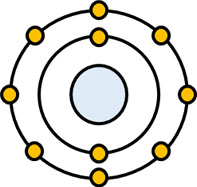But this is not correct. Unless we forget this image, we cannot properly understand electron orbitals, such as s orbitals and p orbitals.

An electron orbital is the probability of an electron’s existence. For example, in a hydrogen atom, the K-shell contains an electron. However, it is not clear whether the electrons exist in the K-shell or not. Possibly, the electron may exist in another place different from the K-shell.

But overall, there is a high probability that the electron in the hydrogen atom is in the K-shell.

### s and p Orbitals (d Orbitals) That Exist in Electron Orbitals

Instead of revolving around an atom like a planet, it is better to think of electrons as a cloud. Something like a cloud exists, and electrons exist inside this cloud. Because of the probability of the existence of electrons, in some cases there may be no electrons in the electron orbit.

Electron orbitals (where the probability of the existence of electrons is high) can be classified into s and p orbitals. Each orbital can have two electrons in it.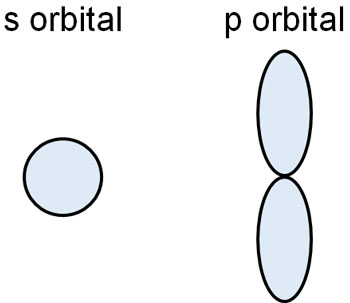The s-orbital is shaped like a sphere. Let’s assume that there is an electron somewhere in the s-orbital (inside the sphere). In the case of molecular hydrogen (H2), two s orbitals are bonded together to form a hydrogen molecule.

On the other hand, the p-orbitals are similar to the number 8. There is only one s-orbital, but there are three p-orbitals. Consider that they are divided into three directions, as shown below.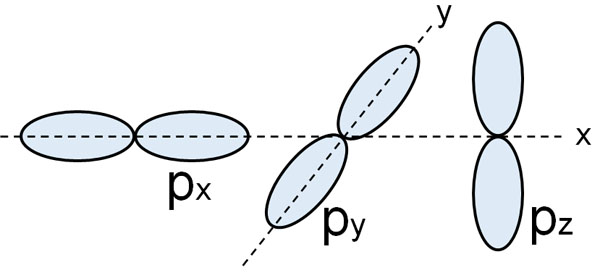The K-shell possesses only s orbital. Therefore, there are two electrons in the s orbital. The L-shell, on the other hand, has one s-orbital and three p-orbitals. A total of eight electrons can be placed in the L-shell.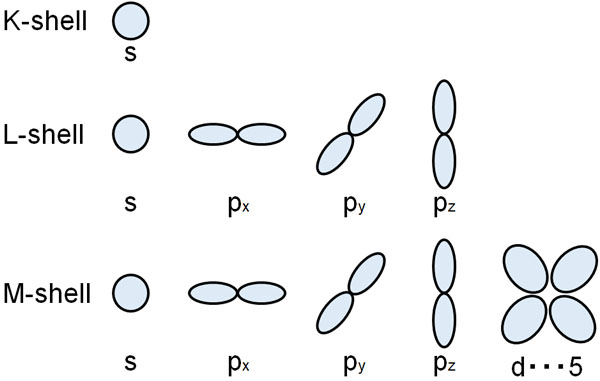Note that in the M-shell, there are not only s and p orbitals, but also d orbitals. However, we rarely consider d-orbitals in organic chemistry, so we only need to understand s and p orbitals at first. Forget about d orbitals, although they do exist.

## What Is Hybridization? How to Identify Hybrid Orbitals and Their Energy

Once you understand the concepts of s and p orbitals, you can move on to the next step in electron orbitals. You can learn about Hybridization.

All matter prefers a stable state. Even human beings prefer to spend time in a resort hotel with a view of the ocean rather than in the middle of the desert. Both humans and electrons prefer to spend time in a stable state rather than an unstable state that requires energy.

When comparing s- and p-orbitals, the energy is lower in the s-orbit. For this reason, electrons first enter the s-orbital instead of the p-orbital. For example, a carbon atom has six electrons. If we consider the order of energy, the electrons enter the s orbital as follows.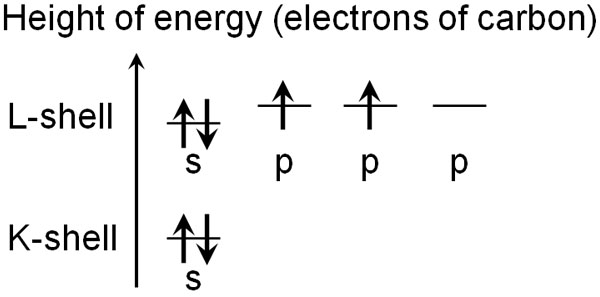But it’s hard to understand in this state: there are differences in energy between s and p orbitals, and electrons don’t exist in the same state.

So, hybrid orbitals is a tool that was invented to make it easier for us to understand, although it doesn’t exist. Essentially, there are s and p orbitals. But instead of these orbitals being mixed, we assume that both s and p orbitals have the same energy and are the same in a hybrid orbitals.

### In Hybrid Orbitals, s and p Orbitals Are Averaged and Considered the Same

By using hybridization, electrons are averaged. For example, a carbon atom has six electrons, so all the orbitals of the L-shell have electrons in them.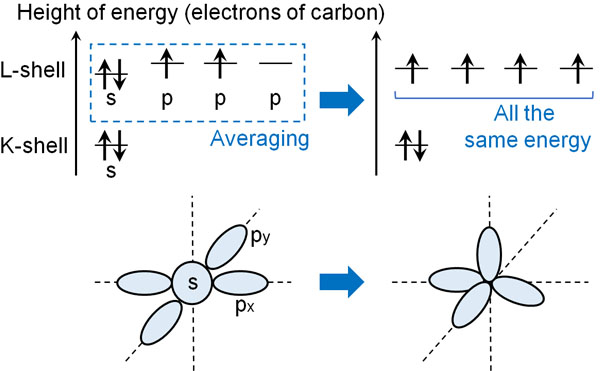When understanding organic compounds, it’s easier to understand them by using hybrid orbitals and considering the s and p orbitals together. Because we assume they are the same, we can eliminate the complexity of the idea.

## The Way to Identify Hybrid Orbitals Is to Count the Number of Hands

This is the concept of s orbital, p orbitals and hybrid orbitals. There is not only one hybridization, but three. They are as follows.

• sp3 hybrid orbitals
• sp2 hybrid orbitals
• sp hybrid orbitals

What is the nature of these hybrid orbitals? They are different in nature and must be clearly identified.

However, it is very easy to distinguish each hybrid orbitals. It can be done by looking at the number of hands. By looking at the number of hands that an atom possesses, we can distinguish the type of hybrid orbitals for a moment. In summary, it is as follows.

 sp3 hybrid orbitals sp2 hybrid orbitals sp hybrid orbitals Four hands three hands two hands

Just understand this. The details of each of these hybrid orbitals will be reviewed below.

### sp3 Hybrid Orbitals: Compounds with Four Hands, Such As Methane and Ethane

Examples of compounds with sp3 hybridization are methane and ethane. In methane and ethane, each carbon atom is bonded to four atoms. Four arms extend from the carbon atoms, each grabbing an atom in its own hand.

Because of the four hands, you can distinguish that the carbon atoms in methane and ethane are sp3 hybrid orbitals.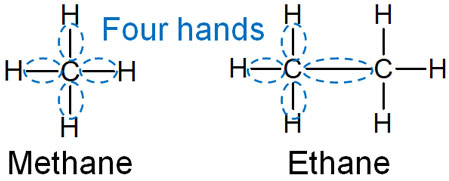In sp3 hybrid orbitals, there is one s-orbital and three p-orbitals. In order to remain stable, each of the four orbitals must point in a different direction. Since the electrons are negatively charged and repel each other, each orbital needs to be at its farthest away from the other.

As a result, the bond angles are 109.5° for the sp3 hybrid orbitals, respectively.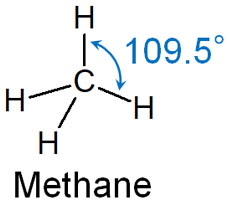The electron orbitals that exist in this way are sp3 hybrid orbitals.

#### Adding an Unshared Electron Pair to the Hand: The Ammonia Example

As a reminder, when identifying hybridization, include the unshared electron pairs. Focus on the unshared electron pairs as well as whether or not they are bound to a particular molecule.

An example is ammonia, which is frequently used as an example. The molecular formula for ammonia is NH3, and it has three hands extending from the nitrogen atom, each grabbing a hydrogen atom; because of the three hands, you would think that it is not an sp3 hybridization.

However, the nitrogen atom has an unshared electron pair. In identifying hybrid orbitals, the unshared electron pairs are also included in the hand. It looks like the following.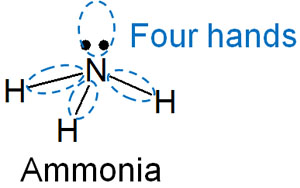Thus, if we include the unshared electron pair, we can see that the nitrogen atom in ammonia has four hands. The reason why ammonia has sp3 hybrid orbital is that it has four hands, including the unshared electron pair.

Since ammonia is an sp3 hybrid orbital, water is also an sp3 hybrid orbital. The molecular formula of water is (H2O). The oxygen atom of water uses two hands to grab the hydrogen atom. In addition to this, there are two unshared electron pairs. Therefore, we can understand that the oxygen atoms of water are sp3 hybrid orbitals.

### sp2 Hybrid Orbitals: Binding Angle of Ethylene (Ethene) and Acetaldehyde

On the other hand, what should we consider for sp2 hybrid orbitals? Compared to sp3 hybrid orbitals, sp2 hybrid orbitals have a smaller number of hands. The number of hands in sp2 hybrid orbitals is three. If an atom has three hands, it is an sp2 hybrid orbital.

For example, sp2 hybrid orbitals are known to include ethylene (ethene), acetaldehyde, formaldehyde, and borane.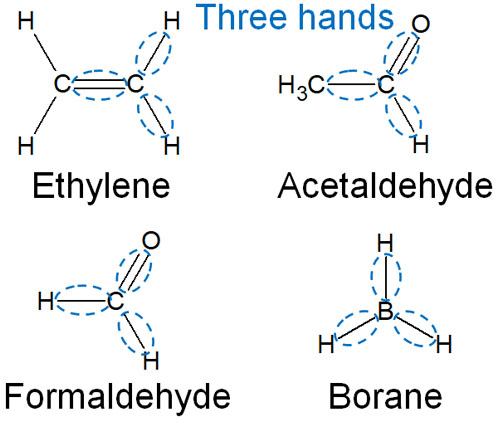If we look at the carbon atom of ethylene, we see that it is bound to other molecules by three hands. The same is true for acetaldehyde, formaldehyde and borane.

In the sp2 hybridizations, they almost have a double bond. There are exceptions, such as borane, which does not have double bonds but has only three hands and is an sp2 hybrid orbital. But in general, you can think of it as forming sp2 hybrid orbitals because of the presence of double bonds.

If the atoms reach out three hands, they are positioned so that they have the most distant bond angles from each other. As a result, the sp2 hybrid orbital has a bonding angle of 120°.Each orbital is most distant and stable at 120°. In any case, understand that sp2 hybridizations are those that are bound to other molecules by three hands.

### sp Hybrid Orbitals: Examples of Acetylene, Acetonitrile and Allene

All hybrid orbitals can be distinguished by the number of hands. sp hybrid orbitals have one less hand than sp2 hybrid orbitals. sp hybrid orbitals have two hands.

In compounds with sp hybrid orbitals, many of them will have double or triple bonds. Examples of sp hybrid orbitals are acetylene, acetonitrile and allene.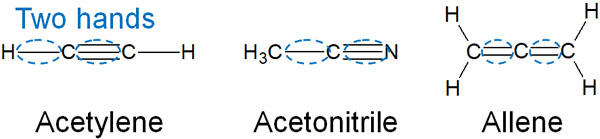There are two hands from the carbon atoms of acetylene. From this situation, we can infer that it is an sp hybrid orbital. The same can be said for acetonitrile and allene.

In order for the two hands to be located at the farthest distance apart, they would have to be at 180° each. If the orbits exist in symmetrical positions, they will be able to take the most stable state.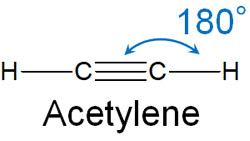Of course, if you look at other atoms they are not sp hybrid orbitals. For example, in acetonitrile, there is a carbon atom with an sp3 hybrid orbital. In the case of allene, there is a carbon atom with an sp2 hybrid orbital. If you focus on different atoms, the type of hybridizations will be different.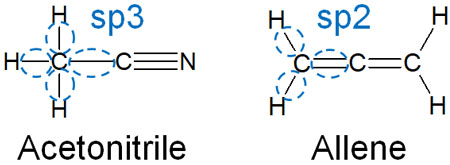Thus, understand that each atom has a different type of hybrid orbital.

Once you learn the concept of these hybridizations, you will be able to distinguish between the hybrid orbitals of all molecules. For example, what are the hybrid orbitals of carbon dioxide? Carbon dioxide (CO2) has the structural formula O=C=O. If we focus on the carbon atoms, we can determine that they are sp hybrid orbitals because they have two hands.

All hybrid orbitals should be determined by how many hands they have.

## Molecular Shape (3D Structure) Is Important in Hybrid Orbitals

So what exactly are these hybrid orbitals? For simplicity, we have focused on the number of hands that each atom has.

Strictly speaking, hybrid orbitals are the shape of molecules. In other words, hybrid orbitals are the elements that determine how the three-dimensional structure is formed.

In the case of sp3 hybrid orbitals, they take the form of a tetrahedron. The bond angle is 109.5°, and the four orbitals take the farthest apart from each other. As a result, the orbits are naturally tetrahedron shaped.

On the other hand, an sp2 hybrid orbital has a bond angle of 120°, and if the three orbitals are the farthest apart, the bond angle is 120°. For sp hybrid orbitals, the opposite position of the molecules results in a bond angle of 180°.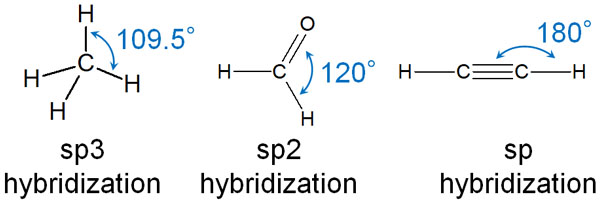These steric structures are determined by the type of hybrid orbitals.

We explained earlier that ammonia is an sp3 hybrid orbital because we need to consider even the unshared electron pairs. However, ammonia has a bonding angle of 107.5°, which is not the ideal bonding angle of 109.5°. Likewise, water (H-O-H) has a bond angle of 104.5°, not 109.5°.

So, strictly speaking, ammonia and water are not sp3 hybrid orbitals. These molecules are not explained by hybrid orbitals. However, it is meaningless to think about them deeply, so we can simply understand that ammonia and water are sp3 hybrid orbitals, including their unshared electron pairs.

## Learn About s and p Orbitals, and Understand the Hybridization of Electrons

There are many people who have graduated from high school chemistry and want to learn more about chemistry. The s-orbitals and p-orbitals are the first concepts learned in any organic chemistry textbook. You will also learn hybrid orbitals.

However, the concept of electron orbitals is difficult. You need to forget what you learned in high school chemistry and you need to relearn new concepts. Also, there are energy differences between orbitals, and you have to use a non-existent tool called hybrid orbitals.

But if you learn one by one, you will be able to understand even the difficult concept of electron orbitals.

Electron orbitals are probably one of the most difficult concepts to understand in organic chemistry. Nevertheless, you have to learn about electron orbitals and hybrid orbitals at the beginning of your textbook. To avoid hating organic chemistry, make sure that you understand the concept of electron orbitals.